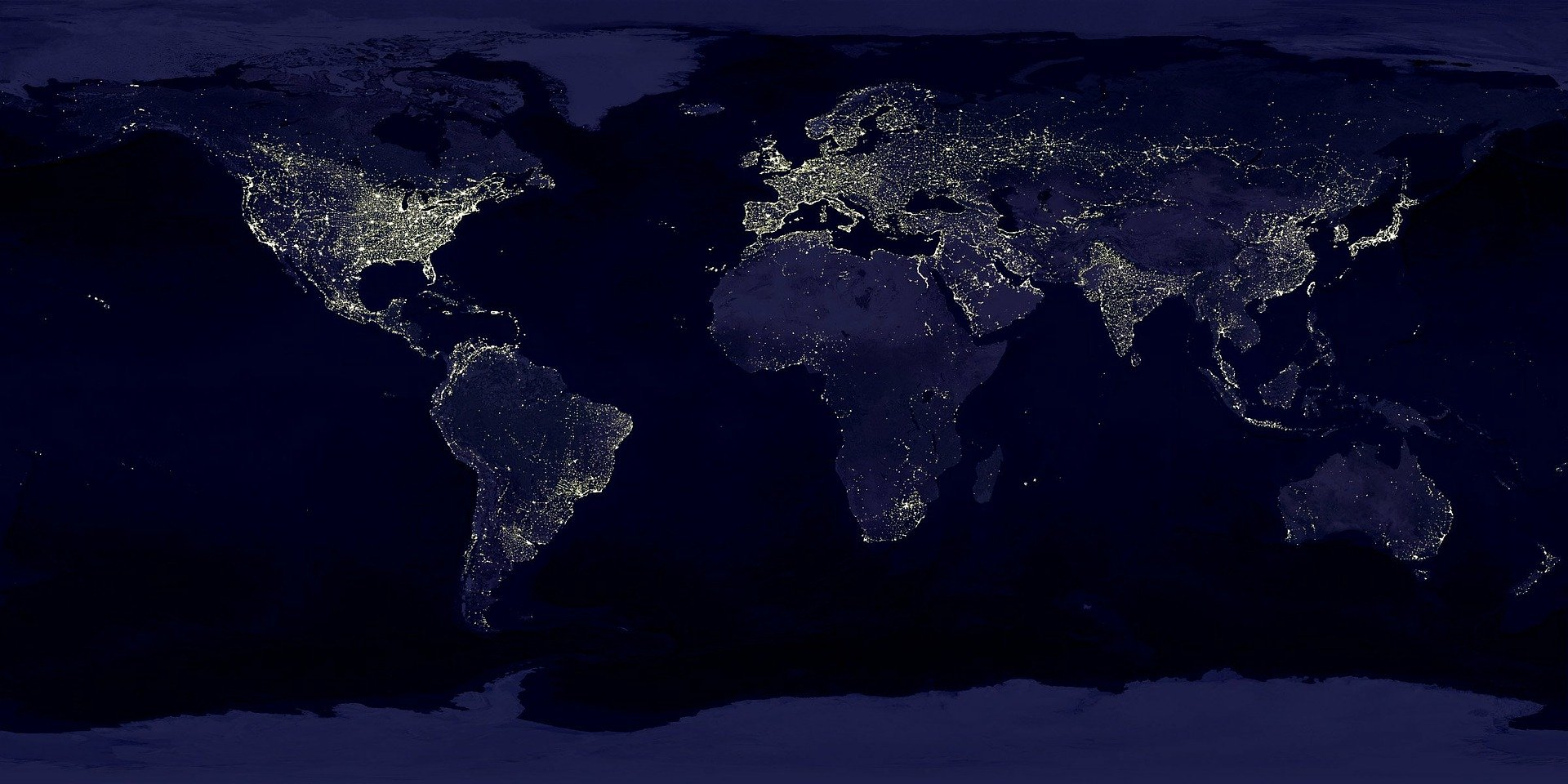April 28, 2020
Engineering

# Converting Geospatial Data in Pandas to MongoDB Geopoint Object

Here is a friendly tutorial to convert geospatial data in pandas DataFrame to MongoDB geospatial object to enable geospatial queries.

There is barely any assistance when it comes to converting Geo-data to a Geopoint object to store in a NoSQL DB, specifically speaking, MongoDB. It was a lot tougher than shooting in the dark, and after wearing the cap of a geeky Holmes the whole night, applying a lot of programmable logic, libraries and a bit of luck, I was able to super power myself to crack it. Here is my blog to help thousands of others who might be needing this help.

Lets look at the basic steps to be followed:

1. Import Libraries
3. Convert Dataframe to GeoDataFrame
4. Use Shapely to convert to GeoJSON
5. DB Connection
6. Convert DF to Dict
7. Test using a Geospatial query

Here are the libraries that you need to do the conversion:

• Pandas
• Geopandas
• Shapely
• Pymongo

I'll walk you through the process of building it, be with me and if you get lost, just start over. But, I got you covered. First, let us start by importing the required libraries and functions.

import pandas as pd
import geopandas
from pymongo import MongoClient,GEOSPHERE
import shapely.geometry

Now, let us read the csv files containing latitude and longitude.

Now, we need to convert the pandas into geopandas dataframe. Doing that is pretty simple.

gdf = geopandas.GeoDataFrame(df, geometry=geopandas.points_from_xy(df.lat, df.lng))
gdf.columns=['lng','lat','loc']

Geodata frame is a pandas dataframe morphed with added geospatial capabilities and coolness. Notice that we have a location column which contains shapely point objects.

Now, we need to convert the shapely point object to a GeoJSON point object. Thankfully, shapely has a built in method to do it.

gdf['loc']=gdf['loc'].apply(lambda x:shapely.geometry.mapping(x))

Shapely's mapping method converts a POINT object to GeoJSON. Since we need this for the whole DataFrame, we will use a lambda function and apply it to the whole location column.

Now, lets create a connection to our local MongoDB instance and create a database and a collection. To enable geospatial queries, we need to use create_index, pass the key name which contains geospatial object and pass 2D_SPHERE as parameter so that MongoDB can recognize 'loc' as a GeoJSON object.

client=MongoClient('localhost',27017)
db = client.geo
collection = db.location
collection.create_index([("loc", GEOSPHERE)])

Now, we shall convert the dataframe to a dictionary since we need data to be in the form of a Key-Value pair in MongoDB. we use orient='records' so that when we want to insert row-wise and have multiple columns. If you want to delete the original lat, lng columns, this is not necessary since there will be only one column left and we will be dealing with a Series object.

data = gdf.to_dict(orient='records')
collection.insert_many(data)
client.close()

We can use insert_many method to bulk insert data. Now, we can close the connection.

We can check our DB to check if its in the right format.

Now, let us run a geospatial query and see if its working.

from pprint import pprint
client=MongoClient('localhost',27017)
db = client.geo
collection = db.location
data=collection.find(
{
"loc": {
"\$near": {
"\$geometry": {
"type": "Point" ,
"coordinates": [64,28]
},
}
}
}).limit(20)

for i in data:
pprint(i)
client.close()

Here is the sample output.

{'_id': ObjectId('5ea8127c34eef174f8c27ca2'),
'lat': 27.981229782104503,
'lng': 64.0141220092773,
'loc': {'coordinates': [64.0141220092773, 27.981229782104503],
'type': 'Point'}}
{'_id': ObjectId('5ea8127c34eef174f8c27c81'),
'lat': 28.0795364379883,
'lng': 64.02834320068361,
'loc': {'coordinates': [64.02834320068361, 28.0795364379883], 'type': 'Point'}}
{'_id': ObjectId('5ea8127c34eef174f8c27b64'),
'lat': 27.9915714263916,
'lng': 63.87321472167971,
'loc': {'coordinates': [63.87321472167971, 27.9915714263916], 'type': 'Point'}}
{'_id': ObjectId('5ea8127c34eef174f8c27c1e'),
'lat': 27.883060455322298,
'lng': 63.999851226806605,
'loc': {'coordinates': [63.999851226806605, 27.883060455322298],
'type': 'Point'}}
...